### Home > A2C > Chapter 9 > Lesson 9.3.2 > Problem9-164

9-164.
1. Change each angle from degrees to radians. Homework Help ✎

1. 60°

2. 75°

3. 210°

4. 225°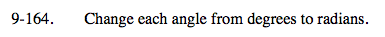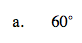$\text{Multiply the angle by }\frac{\pi}{180}.$

$\frac{\pi}{3}$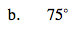See part (a).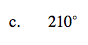See part (a).

$\frac{7\pi}{6}$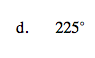See part (a).